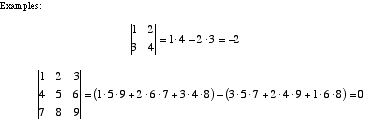index: click on a letter A B C D E F G H I J K L M N O P Q R S T U V W X Y Z A to Z index index: subject areas numbers & symbols sets, logic, proofs geometry algebra trigonometry advanced algebra & pre-calculus calculus advanced topics probability & statistics real world applications multimedia entrieswww.mathwords.com about mathwords website feedback

 Determinant A single number obtained from a matrix that reveals a variety of the matrix's properties. Determinants of small matrices are written and evaluated as shown below. Determinants may also be found using expansion by cofactors. Note: Although a determinant looks like an absolute value it is not. The determinant of a matrix may be negative or positive.this page updated 19-jul-17 Mathwords: Terms and Formulas from Algebra I to Calculus written, illustrated, and webmastered by Bruce Simmons Copyright © 2000 by Bruce Simmons All rights reserved